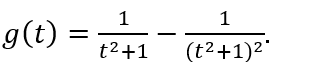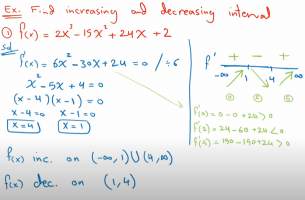How can I solve this e.q

3d904533cd

New memberI need when g(t) is increasing and decreasing

also how can I find local points their if it max or min ?

This one is a bit tricky
I can solve a normal e.q by

getting the derivative then getting the stationary points

for exbut this isn't working what I'm doing wrong ?

Subhotosh Khan

Super Moderator
Staff member
getting the derivative then getting the stationary points
Correct method.

However, we cannot tell you WHY it is not working - because you did not show any work!

What did you get for (dg/dt) ?

3d904533cd

New member
I tried the power rule derivative method

t^2/(t^2+1)^2 = 2t/4t(t^2+1)

Dr.Peterson

Elite Member
I tried the power rule derivative method

t^2/(t^2+1)^2 = 2t/4t(t^2+1)
Have you not learned the quotient rule? You can't just take the derivative of the numerator and denominator separately.

skeeter

Elite Member
I tried the power rule derivative method

t^2/(t^2+1)^2 = 2t/4t(t^2+1)

see post #8